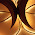### Time and Work -Competitive Exam Questions and Answers With ShortCuts

If you are preparing for competitive examinations, one of the sure shot questions in aptitude is problems related to time and work. Though the questions are simple, without knowing the correct methods, there is a chance of making mistakes. This guide explains various shortcuts which can be used to answer the questions in the work and time section. If you have the correct formula, you can solve most of the questions by simply using it.

For those who are preparing for various government jobs through SSC, PSC and UPSC may find this guide helpful.

Important Formulas and Concepts

• If a man completes certain work in 'n' days, work done by him in one day is 1/n.

• If the work done by a man in one day is 1/n, he can finish the total work in n days.

• Time and work are always inversely proportional to each other.

• If M1 persons can do W1 work in D1 days and M2 persons can do W2 work in D2 days, the general formula to find the total work or total time is :

M1 * D1 * W2 = M2 * D2 * W1

It is important to learn the above concepts to solve problems in Time and Work aptitude questions. Now let us check how to solve these questions using shortcuts.

Solved Examples

1. If A can finish a specific work in 10 days and B can do the same work in 20 days. Find the time required to complete the work by both A and B together

Ans:
```Work was done in a day by A = 1/(10)
Work was done by B in a day = 1/(20)

Work done in a day by both A and B together = ```
`1/(10)  + 1/(20) = (2 + 1)/(20) =3/20 `
` `
`{ Here we took the LCM of both denominators. To learn more about it,`
``` click the link below.

L.C.M. & H.C.F. For Aptitude Questions   }

Now apply the  first law provided in this tutorial to find the time needed```
``` to complete the work by both A and B

=> reverse of 3/20 => 20/3 days

```
2. 40 persons make 60 bricks in 3 hours. if 8 persons leave the work, how many bricks will be made by the remaining persons in the next 6 hours?

Ans:
```Here we need to use the formula M1 * D1 * W2 = M2 * D2 * W1
Here M1 = 40 M2= 32 W1 = 60 D1= 3 D2= 6 W2 = ?

M2  * D2 * W1
____________________

W2 =
M1 * D1

=>  W2 = (32 * 6 * 60)/ (40 *3) = 96 bricks

```
3. If X can do a piece of work in 25 days and Y can finish it in 20 days. They worked together for 5 days and then X left. How many days Y will take to complete the work?

Ans:
```Work was done by X in a day = 1/25
Work was done by Y in a day =1/20

Work done by both X and Y in 5 days = 5 * (1/25 + 1/20) = 9/20

Remaining work = 1 - (9/20) =11/20

Y finish 1/20 work in a day. So to finish 11/20 work, Y needs 11 more days.

```